# User:Tohline/Appendix/Ramblings/RiemannB74C692

(Difference between revisions)

# Another S-type Example b74c692

This chapter is an extension of the chapter we have titled, "Riemann Meets COLLADA & Oculus Rift S." In that chapter we used as our first example of a Riemann S-type ellipsoid the model with parameters, (b/a, c/a) = (0.41, 0.385). Here we construct a model with parameters, (b/a, c/a) = (0.74, 0.692). Other closely related chapters are listed below under the heading, "See Also".

## Key Physical Parameters

The model that we have chosen to use in our second successful construction of a COLLADA-based, 3D and interactive animation has the following properties; this model has been selected from Table 2 of our accompanying discussion of Riemann S-type ellipsoids: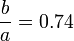$~\frac{b}{a} = 0.74$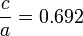$~\frac{c}{a} = 0.692$ Direct Adjoint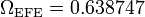$~\Omega_\mathrm{EFE} = 0.638747$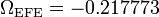$~\Omega_\mathrm{EFE} = - 0.217773$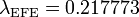$~\lambda_\mathrm{EFE} = 0.217773$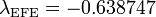$~\lambda_\mathrm{EFE} = - 0.638747$

The subscript "EFE" on Ω and λ means that the relevant frequency is given in units that have been adopted in [EFE], that is, in units of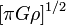$~[\pi G\rho]^{1 / 2}$. In Figure 1a, the solid purple circular marker (where the pair of purple lines cross) identifies the location of this model in the "c/a versus b/a" diagram that appears as Figure 2 on p. 902 of S. Chandrasekhar (1965); essentially the same diagram appears in §49 (p. 147) of [EFE].